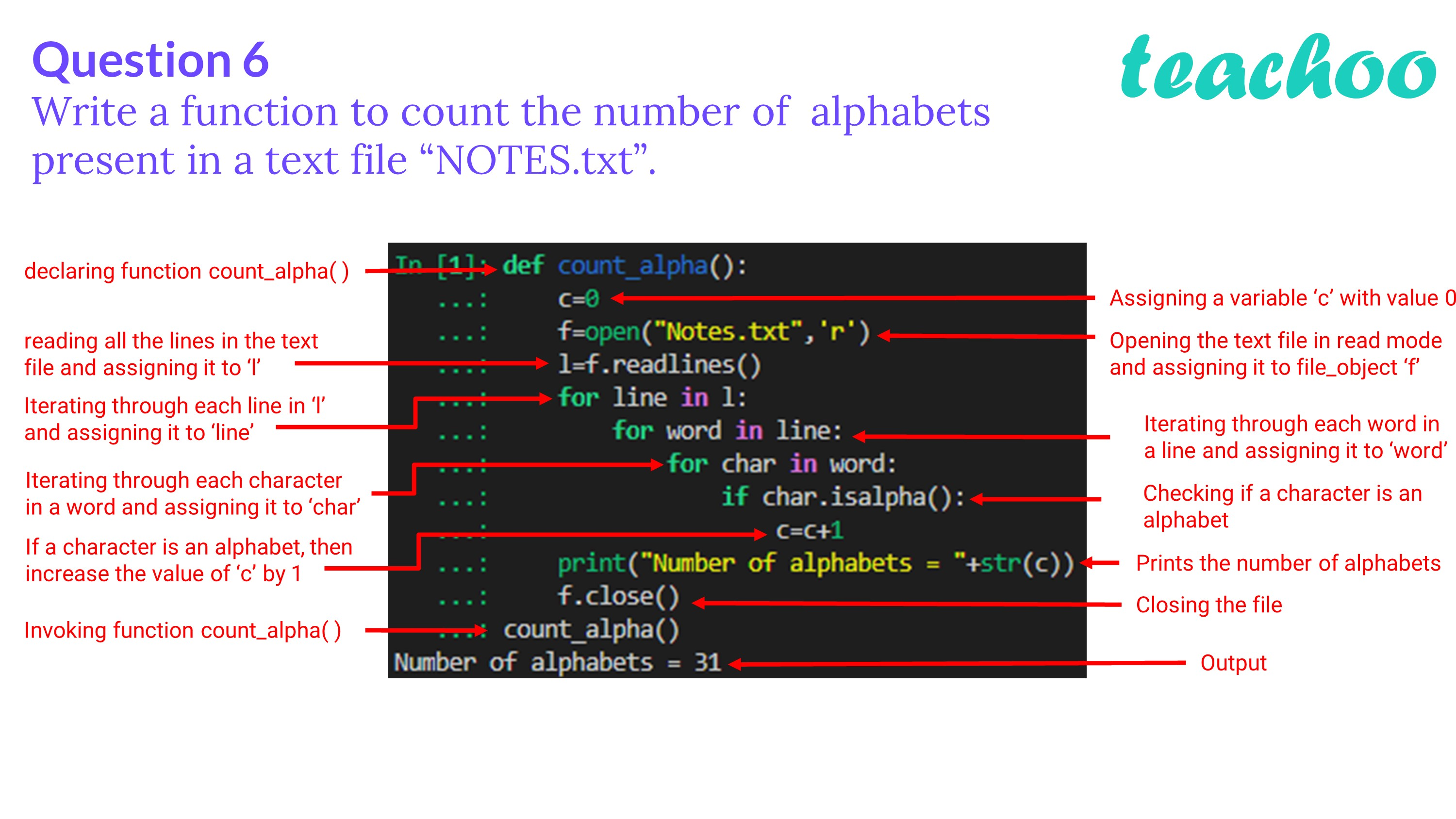Past Year - 2 Mark Questions

Computer Science - Class 12
Chapter 2 - File Handling in Python

## Write a function to count the number of alphabets present in a text file “NOTES.txt”.

``` def count_alpha(): ```

``` c=0 ```

``` f=open("Notes.txt",'r') ```

``` l=f.readlines() ```

``` for line in l: ```

``` for word in line: ```

``` for char in word: ```

``` if char.isalpha(): ```

``` c=c+1 ```

``` print("Number of alphabets = "+str(c)) ```

``` f.close() ```Learn in your speed, with individual attention - Teachoo Maths 1-on-1 Class

### Transcript

Question 6 Write a function to count the number of alphabets present in a text file “NOTES.txt”. declaring function count_alpha( ) reading all the lines in the text file and assigning it to ‘l’ Iterating through each line in ‘l’ and assigning it to ‘line’ Iterating through each character in a word and assigning it to ‘char’ If a character is an alphabet, then increase the value of ‘c’ by 1 Invoking function count_alpha( ) Assigning a variable ‘c’ with value 0 Opening the text file in read mode and assigning it to file_object ‘f’ Iterating through each word in a line and assigning it to ‘word’ Checking if a character is an alphabet Prints the number of alphabets Closing the file Output